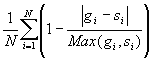# python+opencv识别两张相似图片1.缩放图片，一般大小为8*8，64个像素值。
2.转化为灰度图
3.计算平均值：计算进行灰度处理后图片的所有像素点的平均值，直接用numpy中的mean()计算即可。
4.比较像素灰度值：遍历灰度图片每一个像素，如果大于平均值记录为1，否则为0.
5.得到信息指纹：组合64个bit位，顺序随意保持一致性。

dhash算法

# -*- coding: utf-8 -*-
#feimengjuan
# 利用python实现多种方法来实现图像识别
import cv2
import numpy as np
from matplotlib import pyplot as plt
# 最简单的以灰度直方图作为相似比较的实现
def classify_gray_hist(image1,image2,size = (256,256)):
# 先计算直方图
# 几个参数必须用方括号括起来
# 这里直接用灰度图计算直方图，所以是使用第一个通道，
# 也可以进行通道分离后，得到多个通道的直方图
# bins 取为16
image1 = cv2.resize(image1,size)
image2 = cv2.resize(image2,size)
hist1 = cv2.calchist([image1],,none,,[0.0,255.0])
hist2 = cv2.calchist([image2],,none,,[0.0,255.0])
# 可以比较下直方图
plt.plot(range(256),hist1,’r’)
plt.plot(range(256),hist2,’b’)
plt.show()
# 计算直方图的重合度
degree = 0
for i in range(len(hist1)):
if hist1[i] != hist2[i]:
degree = degree + (1 – abs(hist1[i]-hist2[i])/max(hist1[i],hist2[i]))
else:
degree = degree + 1
degree = degree/len(hist1)
return degree
# 计算单通道的直方图的相似值
def calculate(image1,image2):
hist1 = cv2.calchist([image1],,none,,[0.0,255.0])
hist2 = cv2.calchist([image2],,none,,[0.0,255.0])
# 计算直方图的重合度
degree = 0
for i in range(len(hist1)):
if hist1[i] != hist2[i]:
degree = degree + (1 – abs(hist1[i]-hist2[i])/max(hist1[i],hist2[i]))
else:
degree = degree + 1
degree = degree/len(hist1)
return degree
# 通过得到每个通道的直方图来计算相似度
def classify_hist_with_split(image1,image2,size = (256,256)):
# 将图像resize后，分离为三个通道，再计算每个通道的相似值
image1 = cv2.resize(image1,size)
image2 = cv2.resize(image2,size)
sub_image1 = cv2.split(image1)
sub_image2 = cv2.split(image2)
sub_data = 0
for im1,im2 in zip(sub_image1,sub_image2):
sub_data += calculate(im1,im2)
sub_data = sub_data/3
return sub_data
# 平均哈希算法计算
def classify_ahash(image1,image2):
image1 = cv2.resize(image1,(8,8))
image2 = cv2.resize(image2,(8,8))
gray1 = cv2.cvtcolor(image1,cv2.color_bgr2gray)
gray2 = cv2.cvtcolor(image2,cv2.color_bgr2gray)
hash1 = gethash(gray1)
hash2 = gethash(gray2)
return hamming_distance(hash1,hash2)
def classify_phash(image1,image2):
image1 = cv2.resize(image1,(32,32))
image2 = cv2.resize(image2,(32,32))
gray1 = cv2.cvtcolor(image1,cv2.color_bgr2gray)
gray2 = cv2.cvtcolor(image2,cv2.color_bgr2gray)
# 将灰度图转为浮点型，再进行dct变换
dct1 = cv2.dct(np.float32(gray1))
dct2 = cv2.dct(np.float32(gray2))
# 取左上角的8*8，这些代表图片的最低频率
# 这个操作等价于c++中利用opencv实现的掩码操作
# 在python中进行掩码操作，可以直接这样取出图像矩阵的某一部分
dct1_roi = dct1[0:8,0:8]
dct2_roi = dct2[0:8,0:8]
hash1 = gethash(dct1_roi)
hash2 = gethash(dct2_roi)
return hamming_distance(hash1,hash2)
# 输入灰度图，返回hash
def gethash(image):
avreage = np.mean(image)
hash = []
for i in range(image.shape):
for j in range(image.shape):
if image[i,j] > avreage:
hash.append(1)
else:
hash.append(0)
return hash
# 计算汉明距离
def hamming_distance(hash1,hash2):
num = 0
for index in range(len(hash1)):
if hash1[index] != hash2[index]:
num += 1
return num
if __name__ == ‘__main__’: